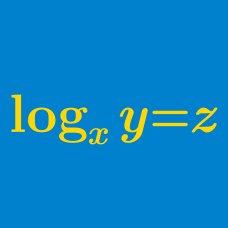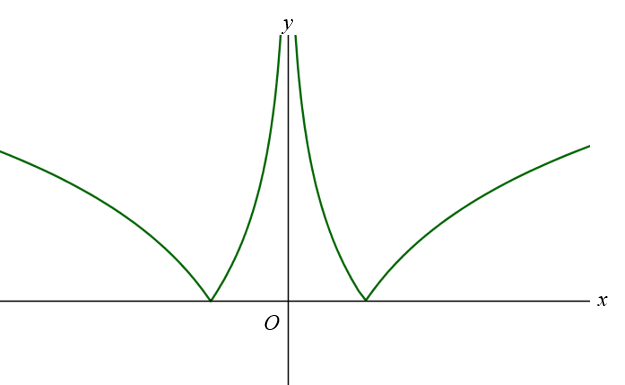Algebra

# Graphs of Logarithmic Functions

Let $f$ be the translation $f: (x,y) \to (x+m, y+n)$, where $m$ and $n$ are constants. If $f$ transforms the curve $y=\log_2 3x$ to $y=\log_2 (12x-108)$, what is $|m+n|$?

Which of the following could be the graph of $\log_a {x} \:$ if $0Which of the following could be the equation of the above graph?

(A) $~ y = \log{|x|}$
(B) $~ y = \left|\log{|x|}\right|$
(C) $~ |y| = \log{x}$
(D) $~ y = |\log{x}|$

For $x$ and $y$ satisfying $x+y=8$, let $m$ be the maximum value of $\log_{4}x+\log_{4}y.$ If $a$ and $b$ are the values of $x$ and $y$, respectively, for which the maximum value is attained, what is $m+2a+3b$?

Which of the following could be the graph of $\log_a {x} \:$ if $0×